GFG App
Open AppBrowser
Continue

# Complete Guide On Array Rotations – Data Structure and Algorithms Tutorial

Rotations in the array is defined as the process of rearranging the elements in an array by shifting each element to a new position. This is mostly done by rotating the elements of the array clockwise or counterclockwise.

## Types of Rotations in Array:

### 1. Right Rotation

Here, The array elements are shifted towards the right.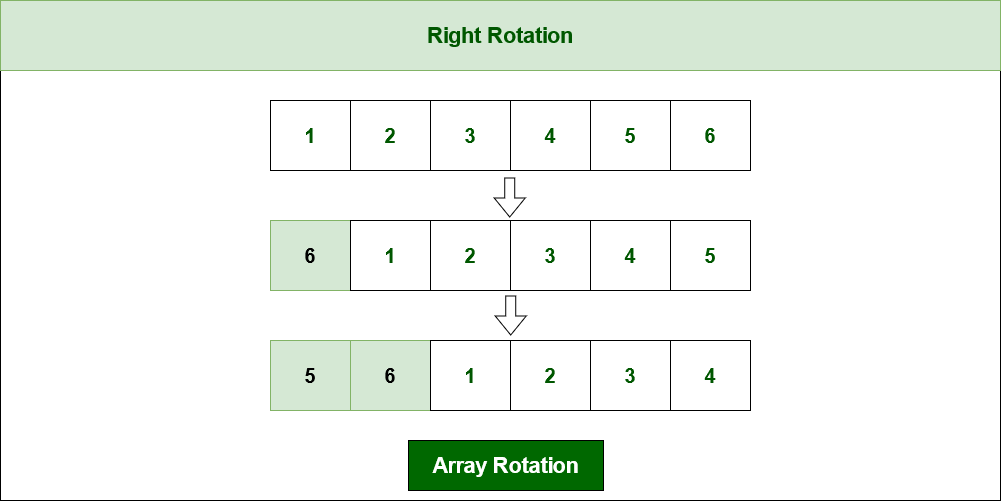Rotating array elements towards right by 2 places

### 2. Left Rotation

Here, The array elements are shifted towards the left.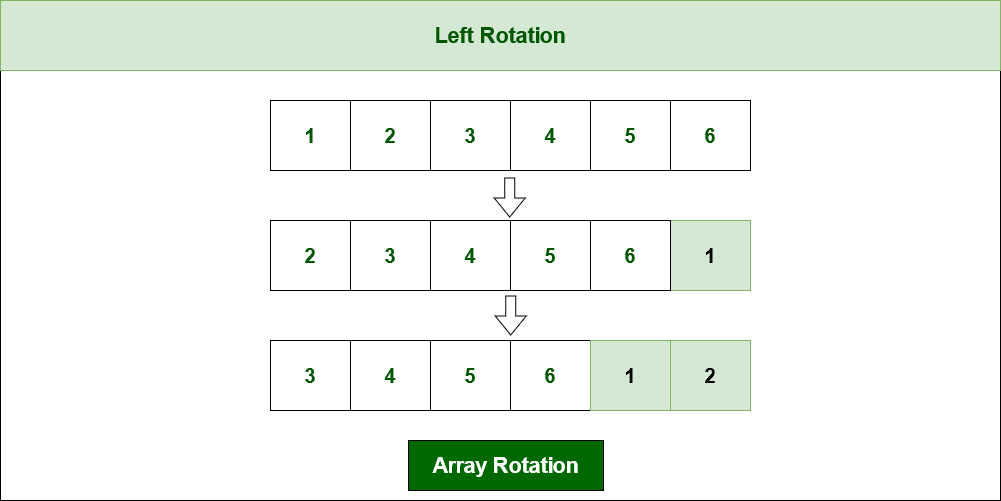Rotating array elements towards left by 2 places

## How to implement rotations in an Array?

There are several ways to implement array rotations. Some of the approaches are mentioned below.

### 1:  Rotate one by one

Here we are considering left rotation. The movements will be just the opposite for right rotation.

Intuition:

At each iteration, shift the elements by one position to the left circularly (i.e., first element becomes the last).
Perform this operation d times to rotate the elements to the left by d position.

Illustration:

Let us take arr[] = [1, 2, 3, 4, 5, 6], d = 2.

First Step:
=> Rotate to left by one position.
=> arr[] = {2, 3, 4, 5, 6, 1}
Second Step:
=> Rotate again to left by one position
=> arr[] = {3, 4, 5, 6, 1, 2}
Rotation is done 2 times.
So the array becomes arr[] = {3, 4, 5, 6, 1, 2}

Below are the steps to solve using the above approach:

• Rotate the array to left by one position. For that do the following:
• Store the first element of the array in a temporary variable.
• Shift the rest of the elements in the original array by one place.
• Update the last index of the array with the temporary variable.
• Repeat the above steps for the number of left rotations required.

Below is the implementation of the above approach:

## C++

 `// C++ code to implement the above approach` `#include ` `using` `namespace` `std;`   `/*Function to left rotate arr[] of size n by d*/` `void` `rotate(``int` `arr[], ``int` `d, ``int` `n)` `{` `    ``int` `p = 1;` `    ``while` `(p <= d) {` `        ``int` `last = arr;` `        ``for` `(``int` `i = 0; i < n - 1; i++) {` `            ``arr[i] = arr[i + 1];` `        ``}` `        ``arr[n - 1] = last;` `        ``p++;` `    ``}` `}`   `// Function to print an array` `void` `printArray(``int` `arr[], ``int` `size)` `{` `    ``for` `(``int` `i = 0; i < size; i++)` `        ``cout << arr[i] << ``" "``;` `}`   `// Driver's code` `int` `main()` `{` `    ``int` `arr[] = { 1, 2, 3, 4, 5, 6 };` `    ``int` `n = ``sizeof``(arr) / ``sizeof``(arr);` `    ``int` `d = 2;`   `    ``// Function calling` `    ``rotate(arr, d, n);` `    ``printArray(arr, n);`   `    ``return` `0;` `}`

## Java

 `// Java code to implement the above approach` `import` `java.io.*;`   `class` `GFG {`   `    ``public` `static` `void` `rotate(``int` `arr[], ``int` `d, ``int` `n)` `    ``{` `        ``int` `p = ``1``;` `        ``while` `(p <= d) {` `            ``int` `last = arr[``0``];` `            ``for` `(``int` `i = ``0``; i < n - ``1``; i++) {` `                ``arr[i] = arr[i + ``1``];` `            ``}` `            ``arr[n - ``1``] = last;` `            ``p++;` `        ``}`   `        ``for` `(``int` `i = ``0``; i < n; i++) {` `            ``System.out.print(arr[i] + ``" "``);` `        ``}` `    ``}`   `    ``// Driver's code` `    ``public` `static` `void` `main(String[] args)` `    ``{` `        ``int` `arr[] = { ``1``, ``2``, ``3``, ``4``, ``5``, ``6` `};` `        ``int` `n = arr.length;` `        ``// Rotate 2 times` `        ``int` `d = ``2``;`   `        ``// Function call` `        ``rotate(arr, d, n);` `    ``}` `}`

## Python3

 `# Python program to implement the above approach`   `# Function to left rotate arr[] of size n by d`     `def` `rotate(arr, d, n):` `    ``p ``=` `1` `    ``while``(p <``=` `d):` `        ``last ``=` `arr[``0``]` `        ``for` `i ``in` `range``(n ``-` `1``):` `            ``arr[i] ``=` `arr[i ``+` `1``]` `        ``arr[n ``-` `1``] ``=` `last` `        ``p ``=` `p ``+` `1`   `# Function to print an array`     `def` `printArray(arr, size):` `    ``for` `i ``in` `range``(size):` `        ``print``(arr[i], end``=``" "``)`     `# Driver code` `arr ``=` `[``1``, ``2``, ``3``, ``4``, ``5``, ``6``]` `n ``=` `len``(arr)` `d ``=` `2`   `# Function calling` `rotate(arr, d, n)` `printArray(arr, n)`

## Javascript

 `function` `rotateArray(arr, d, n) {` `for` `(let i = 0; i < d; i++) {` `const first = arr.shift();` `arr.push(first);` `}` `}`   `// Function to print an array` `function` `printArray(arr) {` `console.log(arr.join(``' '``));` `}`   `// Driver's code` `const arr = [1, 2, 3, 4, 5, 6];` `const n = arr.length;` `const d = 2;`   `// Function calling` `rotateArray(arr, d, n);` `printArray(arr);`

## C#

 `// C# code to implement the above approach` `using` `System;`   `class` `GFG {` `    ``/* Function to left rotate arr[] of size n by d */` `    ``static` `void` `Rotate(``int``[] arr, ``int` `d, ``int` `n) {` `        ``int` `p = 1;` `        ``while` `(p <= d) {` `            ``int` `last = arr;` `            ``for` `(``int` `i = 0; i < n - 1; i++) {` `                ``arr[i] = arr[i + 1];` `            ``}` `            ``arr[n - 1] = last;` `            ``p++;` `        ``}` `    ``}` `    ``// Function to print an array` `    ``static` `void` `PrintArray(``int``[] arr, ``int` `size) {` `        ``for` `(``int` `i = 0; i < size; i++)` `            ``Console.Write(arr[i] + ``" "``);` `    ``}`   `    ``// Driver's code` `    ``static` `void` `Main(``string``[] args) {` `        ``int``[] arr = { 1, 2, 3, 4, 5, 6 };` `        ``int` `n = arr.Length;` `        ``int` `d = 2;`   `        ``// Function calling` `        ``Rotate(arr, d, n);` `        ``PrintArray(arr, n);` `    ``}` `}`

Output

`3 4 5 6 1 2 `

Time Complexity: O(N * d)
Auxiliary Space: O(1)

### 2. Using temporary Array:

Here we are considering the right rotation. The movements will be just the opposite for left rotations.

Intuition:

In this technique, create a temporary array of size n, where n is the length of the original array. If we rotate the array by d positions to right, the last d elements will be in the front. So, copy the last d elements of the original array into the first d positions of the temporary array and copy the remaining elements in the remaining positions. Finally, copy the elements of the temporary array back into the original array starting at position 0.

Illustration:

• Initialize an empty temp array, to store the final rotated state of the original array.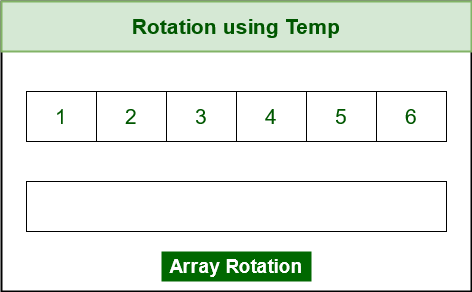Example of array

• Copy all elements from (n-d) to the end of the original to the front of the temp array.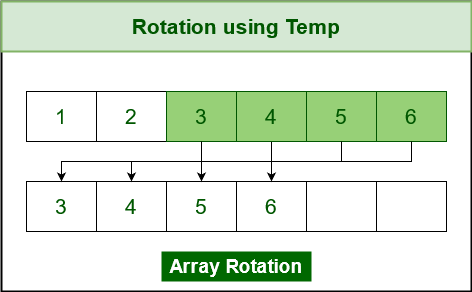Copy last ‘d’ elements to the front in temp[]

• Now copy the rest elements from the front to the back of the temp[].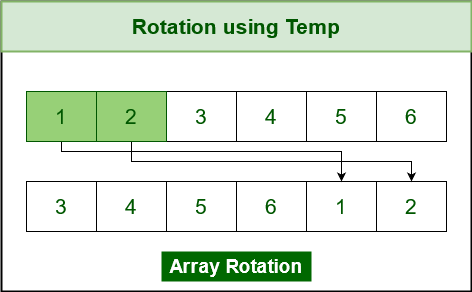Copy the remaining elements to the end

Below are the steps to solve using the above approach:

• Initializing a temporary array (temp[n]) of length same as the original array.
• Initialize ( j ) to keep track of the current index.
• Store elements from position d to n-1 in the temp array.
• Now, store 0 to d-1 in the temp array from the original array.
• Lastly, copy back temp[] to the original as the final output.

Below is the implementation of the above approach

## C++

 `// C++ code to implement the above approach` `#include ` `using` `namespace` `std;`   `// Function to rotate array` `void` `rotate(``int` `arr[], ``int` `d, ``int` `n)` `{` `    ``// Storing rotated version of array` `    ``int` `temp[n];`   `    ``// Keeping track of the current index` `    ``// of temp[]` `    ``int` `j = 0;`   `    ``// Storing the n - d elements of` `    ``// array arr[] to the front of temp[]` `    ``for` `(``int` `i = d; i < n; i++) {` `        ``temp[j] = arr[i];` `        ``j++;` `    ``}`   `    ``// Storing the first d elements of array arr[]` `    ``//  into temp` `    ``for` `(``int` `i = 0; i < d; i++) {` `        ``temp[j] = arr[i];` `        ``j++;` `    ``}`   `    ``// Copying the elements of temp[] in arr[]` `    ``// to get the final rotated array` `    ``for` `(``int` `i = 0; i < n; i++) {` `        ``arr[i] = temp[i];` `    ``}` `}`   `// Function to print elements of array` `void` `printTheArray(``int` `arr[], ``int` `n)` `{` `    ``for` `(``int` `i = 0; i < n; i++) {` `        ``cout << arr[i] << ``" "``;` `    ``}` `}`   `// Driver's code` `int` `main()` `{` `    ``int` `arr[] = { 1, 2, 3, 4, 5, 6 };` `    ``int` `N = ``sizeof``(arr) / ``sizeof``(arr);` `    ``int` `d = 2;`   `    ``// Function calling` `    ``rotate(arr, d, N);` `    ``printTheArray(arr, N);`   `    ``return` `0;` `}`

## Java

 `// Java code to implement the above approach` `import` `java.io.*;`   `class` `GFG {`   `    ``// Function to rotate array` `    ``static` `void` `rotate(``int` `arr[], ``int` `d, ``int` `n)` `    ``{` `        ``// Storing rotated version of array` `        ``int` `temp[] = ``new` `int``[n];`   `        ``// Keeping track of the current index` `        ``// of temp[]` `        ``int` `j = ``0``;`   `        ``// Storing the n - d elements of` `        ``// array arr[] to the front of temp[]` `        ``for` `(``int` `i = d; i < n; i++) {` `            ``temp[j] = arr[i];` `            ``j++;` `        ``}`   `        ``// Storing the first d elements of array arr[]` `        ``// into temp` `        ``for` `(``int` `i = ``0``; i < d; i++) {` `            ``temp[j] = arr[i];` `            ``j++;` `        ``}`   `        ``// Copying the elements of temp[] in arr[]` `        ``// to get the final rotated array` `        ``for` `(``int` `i = ``0``; i < n; i++) {` `            ``arr[i] = temp[i];` `        ``}` `    ``}`   `    ``// Function to print elements of array` `    ``static` `void` `printTheArray(``int` `arr[], ``int` `n)` `    ``{` `        ``for` `(``int` `i = ``0``; i < n; i++) {` `            ``System.out.print(arr[i] + ``" "``);` `        ``}` `    ``}` `    ``public` `static` `void` `main(String[] args)` `    ``{` `        ``int` `arr[] = { ``1``, ``2``, ``3``, ``4``, ``5``, ``6` `};` `        ``int` `N = arr.length;` `        ``int` `d = ``2``;`   `        ``// Function calling` `        ``rotate(arr, d, N);` `        ``printTheArray(arr, N);` `    ``}` `}`

## Python3

 `# Python code to implement the above approach` `def` `rotate(L, d, n):` `    ``k ``=` `L.index(d)` `    ``new_lis ``=` `[]` `    ``new_lis ``=` `L[k``+``1``:]``+``L[``0``:k``+``1``]` `    ``return` `new_lis`     `if` `__name__ ``=``=` `'__main__'``:` `    ``arr ``=` `[``1``, ``2``, ``3``, ``4``, ``5``, ``6``]` `    ``d ``=` `2` `    ``N ``=` `len``(arr)`   `    ``# Function call` `    ``arr ``=` `rotate(arr, d, N)` `    ``for` `i ``in` `arr:` `        ``print``(i, end``=``" "``)`

## Javascript

 `function` `rotateArray(arr, d, n) {` `// Storing rotated version of array` `let temp = [];`   `// Keeping track of the current index` `// of temp[]` `let j = 0;`   `// Storing the n - d elements of` `// array arr[] to the front of temp[]` `for` `(let i = d; i < n; i++) {` `temp[j] = arr[i];` `j++;` `}`   `// Storing the first d elements of array arr[]` `// into temp` `for` `(let i = 0; i < d; i++) {` `temp[j] = arr[i];` `j++;` `}`   `// Copying the elements of temp[] in arr[]` `// to get the final rotated array` `for` `(let i = 0; i < n; i++) {` `arr[i] = temp[i];` `}` `}`   `// Function to print elements of array` `function` `printArray(arr) {` `console.log(arr.join(``' '``));` `}`   `// Driver's code` `let arr = [1, 2, 3, 4, 5, 6];` `let N = arr.length;` `let d = 2;`   `// Function calling` `rotateArray(arr, d, N);` `printArray(arr);`

## C#

 `// C# code for the approach`   `using` `System;`   `class` `GFG {` `      ``// Driver code` `    ``static` `void` `Main(``string``[] args)` `    ``{` `          ``// Input array` `        ``int``[] arr = { 1, 2, 3, 4, 5, 6 };` `        ``int` `N = arr.Length;` `        ``int` `d = 2;` `      `  `        ``// Storing rotated version of array` `        ``int``[] temp = ``new` `int``[N];`   `        ``// Keeping track of the current index` `        ``// of temp[]` `        ``int` `j = 0;`   `        ``// Storing the n - d elements of` `        ``// array arr[] to the front of temp[]` `        ``for` `(``int` `i = d; i < N; i++) {` `            ``temp[j] = arr[i];` `            ``j++;` `        ``}`   `        ``// Storing the first d elements of array arr[]` `        ``// into temp` `        ``for` `(``int` `i = 0; i < d; i++) {` `            ``temp[j] = arr[i];` `            ``j++;` `        ``}`   `        ``// Copying the elements of temp[] in arr[]` `        ``// to get the final rotated array` `        ``for` `(``int` `i = 0; i < N; i++) {` `            ``arr[i] = temp[i];` `        ``}`   `        ``// Function to print elements of array` `        ``foreach``(``int` `element ``in` `arr)` `        ``{` `            ``Console.Write(element + ``" "``);` `        ``}` `    ``}` `}`

Output

`3 4 5 6 1 2 `

Time complexity: O(N)
Auxiliary Space: O(N)

### 3:  Juggling Algorithm

Intuition:

Instead of moving one by one, divide the array into different sets where the number of sets is equal to the GCD of n and d (say x. So the elements which are x distance apart are part of a set) and rotate the elements within sets by 1 position to the left.

Calculate the GCD between the length and the distance to be moved.
The elements are only shifted within the sets.
We start with temp = arr and keep moving arr[I+d] to arr[I] and finally store temp at the right place.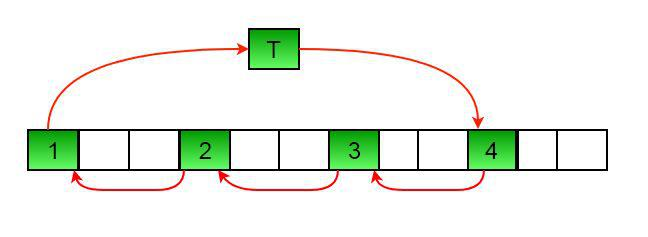Juggling Algorithm

Illustration:

Let arr[] = {0, 1, 2, 3, 4, 5, 6, 7, 8, 9, 10, 11, 12, 13, 14} and d = 10
First step:
=> First set is {0, 5, 10}.
=> Rotate this set by d position in cyclic order
=> arr = arr[0+10]
=> arr = arr[(10+10)%15]
=> arr = arr
=> This set becomes {10,0,5}
=> Array arr[] = {10, 1, 2, 3, 4, 0, 6, 7, 8, 9, 5, 11, 12, 13, 14}
Second step:
=> Second set is {1, 6, 11}.
=> Rotate this set by d position in cyclic order.
=> This set becomes {11, 1, 6}
=> Array arr[] =  {10, 11, 2, 3, 4, 0, 1, 7, 8, 9, 5, 6, 12, 13, 14}
Third step:
=> Second set is {2, 7, 12}.
=> Rotate this set by d position in cyclic order.
=> This set becomes {12, 2, 7}
=> Array arr[] =  {10, 11, 12, 3, 4, 0, 1, 2, 8, 9, 5, 6, 7, 13, 14}
Fourth step:
=> Second set is {3, 8, 13}.
=> Rotate this set by d position in cyclic order.
=> This set becomes {13, 3, 8}
=> Array arr[] =  {10, 11, 12, 13, 4, 0, 1, 2, 3, 9, 5, 6, 7, 8, 14}
Fifth step:
=> Second set is {4, 9, 14}.
=> Rotate this set by d position in cyclic order.
=> This set becomes {14, 4, 9}
=> Array arr[] =  {10, 11, 12, 13, 14, 0, 1, 2, 3, 4, 5, 6, 7, 8, 9}

Below are the steps to solve using the above approach:

• Perform d%n in order to keep the value of d within the range of the array where d is the number of times the array is rotated and n is the size of the array.
• Calculate the GCD(n, d) to divide the array into sets.
• Run a for loop from 0 to the value obtained from GCD.
• Store the value of arr[i] in a temporary variable (the value of i denotes the set number).
• Run a while loop to update the values according to the set.
• After exiting the while loop assign the value of arr[j] as the value of the temporary variable (the value of j denotes the last element of the ith set).

Below is the implementation of the above approach:

## C++

 `// C++ program to implement the above approach` `#include ` `using` `namespace` `std;`   `/*Function to get gcd of a and b*/` `int` `gcd(``int` `a, ``int` `b)` `{` `    ``if` `(b == 0)` `        ``return` `a;`   `    ``else` `        ``return` `gcd(b, a % b);` `}`   `/*Function to left rotate arr[] of size n by d*/` `void` `leftRotate(``int` `arr[], ``int` `d, ``int` `n)` `{` `    ``/* To handle if d >= n */` `    ``d = d % n;` `    ``int` `g_c_d = gcd(d, n);` `    ``for` `(``int` `i = 0; i < g_c_d; i++) {` `        ``/* move i-th values of blocks */` `        ``int` `temp = arr[i];` `        ``int` `j = i;`   `        ``while` `(1) {` `            ``int` `k = j + d;` `            ``if` `(k >= n)` `                ``k = k - n;`   `            ``if` `(k == i)` `                ``break``;`   `            ``arr[j] = arr[k];` `            ``j = k;` `        ``}` `        ``arr[j] = temp;` `    ``}` `}`   `// Function to print an array` `void` `printArray(``int` `arr[], ``int` `size)` `{` `    ``for` `(``int` `i = 0; i < size; i++)` `        ``cout << arr[i] << ``" "``;` `}`   `// Driver's code` `int` `main()` `{` `    ``int` `arr[] = { 1, 2, 3, 4, 5, 6 };` `    ``int` `n = ``sizeof``(arr) / ``sizeof``(arr);` `    ``int` `d = 2;` `    ``// Function calling` `    ``leftRotate(arr, d, n);` `    ``printArray(arr, n);`   `    ``return` `0;` `}`

## Java

 `// Java program to implement the above approach` `import` `java.io.*;` `class` `RotateArray {` `    ``/*Function to left rotate arr[] of siz n by d*/` `    ``void` `leftRotate(``int` `arr[], ``int` `d, ``int` `n)` `    ``{` `        ``/* To handle if d >= n */` `        ``d = d % n;` `        ``int` `i, j, k, temp;` `        ``int` `g_c_d = gcd(d, n);` `        ``for` `(i = ``0``; i < g_c_d; i++) {` `            ``/* move i-th values of blocks */` `            ``temp = arr[i];` `            ``j = i;` `            ``while` `(``true``) {` `                ``k = j + d;` `                ``if` `(k >= n)` `                    ``k = k - n;` `                ``if` `(k == i)` `                    ``break``;` `                ``arr[j] = arr[k];` `                ``j = k;` `            ``}` `            ``arr[j] = temp;` `        ``}` `    ``}`   `    ``/*UTILITY FUNCTIONS*/`   `    ``/* function to print an array */` `    ``void` `printArray(``int` `arr[], ``int` `size)` `    ``{` `        ``int` `i;` `        ``for` `(i = ``0``; i < size; i++)` `            ``System.out.print(arr[i] + ``" "``);` `    ``}`   `    ``/*Function to get gcd of a and b*/` `    ``int` `gcd(``int` `a, ``int` `b)` `    ``{` `        ``if` `(b == ``0``)` `            ``return` `a;` `        ``else` `            ``return` `gcd(b, a % b);` `    ``}`   `    ``// Driver's code` `    ``public` `static` `void` `main(String[] args)` `    ``{` `        ``RotateArray rotate = ``new` `RotateArray();` `        ``int` `arr[] = { ``1``, ``2``, ``3``, ``4``, ``5``, ``6` `};` `        ``int` `n = ``6``;` `        ``int` `d = ``2``;` `        ``rotate.leftRotate(arr, d, n);` `        ``rotate.printArray(arr, n);` `    ``}` `}`

## Python3

 `# Python code to implement the above approach`     `# Function to rotate the array left by d positions` `def` `leftRotate(arr, d, n):` `    ``d ``=` `d ``%` `n` `    ``g_c_d ``=` `gcd(d, n)` `    ``for` `i ``in` `range``(g_c_d):`   `        ``# move i-th values of blocks` `        ``temp ``=` `arr[i]` `        ``j ``=` `i` `        ``while` `1``:` `            ``k ``=` `j ``+` `d` `            ``if` `k >``=` `n:` `                ``k ``=` `k ``-` `n` `            ``if` `k ``=``=` `i:` `                ``break` `            ``arr[j] ``=` `arr[k]` `            ``j ``=` `k` `        ``arr[j] ``=` `temp`     `# function to print an array` `def` `printArray(arr, size):` `    ``for` `i ``in` `range``(size):` `        ``print``(arr[i], end``=``" "``)`     `# Function to get gcd of a and b` `def` `gcd(a, b):` `    ``if` `b ``=``=` `0``:` `        ``return` `a` `    ``else``:` `        ``return` `gcd(b, a ``%` `b)`     `# Driver code` `if` `__name__ ``=``=` `'__main__'``:` `    ``arr ``=` `[``1``, ``2``, ``3``, ``4``, ``5``, ``6``]` `    ``n ``=` `len``(arr)` `    ``d ``=` `2` `    ``leftRotate(arr, d, n)` `    ``printArray(arr, n)`

## C#

 `using` `System;`   `namespace` `LeftRotation` `{` `  ``class` `Program` `  ``{` `    ``// Function to get gcd of a and b` `    ``static` `int` `gcd(``int` `a, ``int` `b)` `    ``{` `      ``if` `(b == 0)` `        ``return` `a;` `      ``else` `        ``return` `gcd(b, a % b);` `    ``}`   `    ``// Function to left rotate arr[] of size n by d` `    ``static` `void` `leftRotate(``int``[] arr, ``int` `d, ``int` `n)` `    ``{` `      ``// To handle if d >= n` `      ``d = d % n;` `      ``int` `g_c_d = gcd(d, n);` `      ``for` `(``int` `i = 0; i < g_c_d; i++)` `      ``{` `        ``// move i-th values of blocks` `        ``int` `temp = arr[i];` `        ``int` `j = i;`   `        ``while` `(``true``)` `        ``{` `          ``int` `k = j + d;` `          ``if` `(k >= n)` `            ``k = k - n;`   `          ``if` `(k == i)` `            ``break``;`   `          ``arr[j] = arr[k];` `          ``j = k;` `        ``}` `        ``arr[j] = temp;` `      ``}` `    ``}`   `    ``// Function to print an array` `    ``static` `void` `printArray(``int``[] arr, ``int` `size)` `    ``{` `      ``for` `(``int` `i = 0; i < size; i++)` `        ``Console.Write(arr[i] + ``" "``);` `    ``}`   `    ``// Driver's code` `    ``static` `public` `void` `Main(``string``[] args)` `    ``{` `      ``int``[] arr = { 1, 2, 3, 4, 5, 6 };` `      ``int` `n = arr.Length;` `      ``int` `d = 2;` `      ``// Function calling` `      ``leftRotate(arr, d, n);` `      ``printArray(arr, n);` `    ``}` `  ``}` `}`

## Javascript

 `function` `gcd(a, b) {` `if` `(b == 0) {` `return` `a;` `} ``else` `{` `return` `gcd(b, a % b);` `}` `}`   `// Function to left rotate arr[] of size n by d` `function` `leftRotate(arr, d, n) {` `// To handle if d >= n` `d = d % n;` `let g_c_d = gcd(d, n);` `for` `(let i = 0; i < g_c_d; i++) {` `// move i-th values of blocks` `let temp = arr[i];` `let j = i;` `while` `(1) {` `let k = j + d;` `if` `(k >= n) {` `k = k - n;` `}` `if` `(k == i) {` `break``;` `}` `arr[j] = arr[k];` `j = k;` `}` `arr[j] = temp;` `}` `}`   `// Function to print an array` `function` `printArray(arr) {` `let str = ``""``;` `for` `(let i = 0; i < arr.length; i++) {` `str += arr[i] + ``" "``;` `}` `console.log(str);` `}`   `// Driver's code` `let arr = [1, 2, 3, 4, 5, 6];` `let n = arr.length;` `let d = 2;`   `// Function calling` `leftRotate(arr, d, n);` `printArray(arr);`

Output

`3 4 5 6 1 2 `

Time complexity : O(N)
Auxiliary Space : O(1)

### 4. The Reversal Algorithm

Intuition:

If observed closely, we can see that a group of array elements is changing its position. For example see the following array:
arr[] = {1, 2, 3, 4, 5, 6} and d = 2. The rotated array is {3, 4, 5, 6, 1, 2}
The group having the first two elements is moving to the end of the array. This is like reversing the array.
But the issue is that if we only reverse the array, it becomes { 6, 5, 4, 3, 2, 1}.
After rotation, the elements in the chunks having the first 5 elements { 6, 5, 4, 3} and the last 2 elements {2, 1} should be in the actual order as of the initial array [i.e., {3, 4, 5, 6} and {1, 2}]but here it gets reversed.
So if those blocks are reversed again we get the desired rotated array.
So the sequence of operations is:

• Reverse the whole array
• Then reverse the last ‘d’ elements and
• Then reverse the first (N-d) elements.

As we are performing reverse operations it is also similar to the following sequence:

• Reverse the first ‘d’ elements
• Reverse last (N-d) elements
• Reverse the whole array.

Illustration:

Take the original array as it is.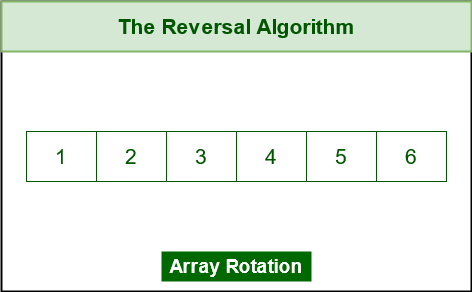Example of Array

• Separate it out into first ‘d’ elements and ‘n-d’ elements.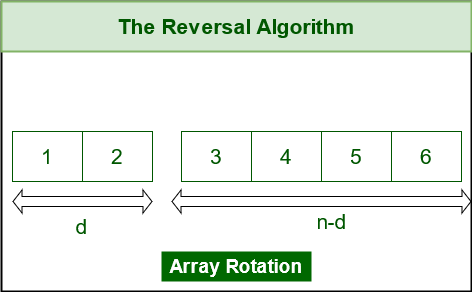Separate array into first ‘d’ and (n-d) segment

• Reverse first ‘d’ elements.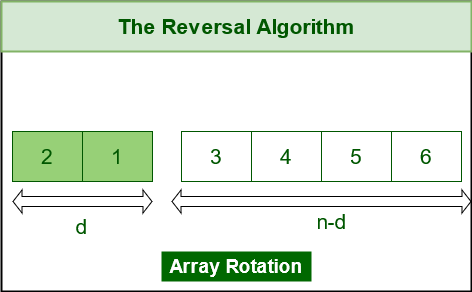Reverse the first ‘d’ elements

• Reverse the next ‘n-d’ elements.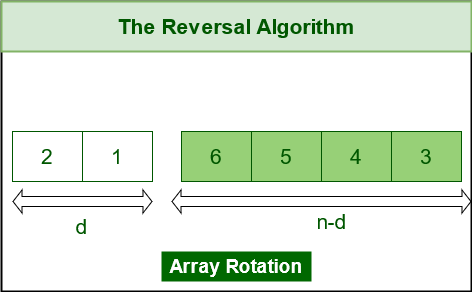Reverse the next (n-d) elements

• Next, reverse the whole array. We have the final array rotated by d elements to left.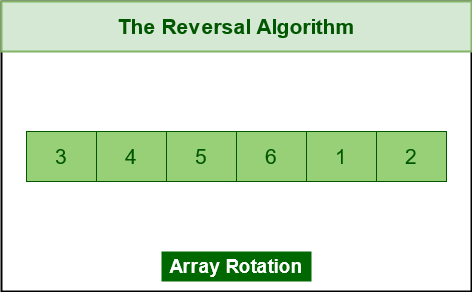Reverse the whole array

Below is the implementation of the above approach:

## C++

 `// C++ code to implement the above approach` `#include `   `using` `namespace` `std;`   `// Function to rotate an array by k elements to the left` `void` `rotateArray(vector<``int``>& arr, ``int` `d)` `{` `    ``// Find the size of the array` `    ``int` `n = arr.size();`   `    ``// Mod k with the size of the array` `    ``// To handle the case where k is greater than the size` `    ``// of the array` `    ``d %= n;`   `    ``// Reverse the first k elements` `    ``reverse(arr.begin(), arr.begin() + d);`   `    ``// Reverse the remaining n-k elements` `    ``reverse(arr.begin() + d, arr.end());`   `    ``// Reverse the entire array` `    ``reverse(arr.begin(), arr.end());` `}`   `int` `main()` `{` `    ``// Initialize the array` `    ``vector<``int``> arr = { 1, 2, 3, 4, 5, 6 };`   `    ``// Number of elements to rotate to the right` `    ``int` `d = 2;`   `    ``// Call the rotateArray function to rotate the array` `    ``rotateArray(arr, d);`   `    ``// Print the rotated array` `    ``for` `(``int` `i : arr) {` `        ``cout << i << ``" "``;` `    ``}`   `    ``// Return 0 to indicate successful termination of the` `    ``// program` `    ``return` `0;` `}`

## Java

 `// Java program to implement the above approach` `import` `java.io.*;`   `class` `LeftRotate {` `    ``/* Function to left rotate arr[] of size n by d */` `    ``static` `void` `leftRotate(``int` `arr[], ``int` `d)` `    ``{`   `        ``if` `(d == ``0``)` `            ``return``;`   `        ``int` `n = arr.length;` `        ``// in case the rotating factor is` `        ``// greater than array length` `        ``d = d % n;` `        ``reverseArray(arr, ``0``, d - ``1``);` `        ``reverseArray(arr, d, n - ``1``);` `        ``reverseArray(arr, ``0``, n - ``1``);` `    ``}`   `    ``/*Function to reverse arr[] from index start to end*/` `    ``static` `void` `reverseArray(``int` `arr[], ``int` `start, ``int` `end)` `    ``{` `        ``int` `temp;` `        ``while` `(start < end) {` `            ``temp = arr[start];` `            ``arr[start] = arr[end];` `            ``arr[end] = temp;` `            ``start++;` `            ``end--;` `        ``}` `    ``}`   `    ``/*UTILITY FUNCTIONS*/` `    ``/* function to print an array */` `    ``static` `void` `printArray(``int` `arr[])` `    ``{` `        ``for` `(``int` `i = ``0``; i < arr.length; i++)` `            ``System.out.print(arr[i] + ``" "``);` `    ``}`   `    ``/* Driver program to test above functions */` `    ``public` `static` `void` `main(String[] args)` `    ``{` `        ``int` `arr[] = { ``1``, ``2``, ``3``, ``4``, ``5``, ``6` `};` `        ``int` `n = arr.length;` `        ``int` `d = ``2``;`   `        ``leftRotate(arr, d); ``// Rotate array by d` `        ``printArray(arr);` `    ``}` `}`

## Python3

 `# Python code to implement the above approach`     `# Function to reverse start to end of array` `def` `reverseArray(arr, start, end):` `    ``while` `(start < end):` `        ``temp ``=` `arr[start]` `        ``arr[start] ``=` `arr[end]` `        ``arr[end] ``=` `temp` `        ``start ``+``=` `1` `        ``end ``=` `end``-``1`     `# Function to left rotate arr[] of size n by d` `def` `leftRotate(arr, d):`   `    ``if` `d ``=``=` `0``:` `        ``return` `    ``n ``=` `len``(arr)` `    ``# in case the rotating factor is` `    ``# greater than array length` `    ``d ``=` `d ``%` `n` `    ``reverseArray(arr, ``0``, d``-``1``)` `    ``reverseArray(arr, d, n``-``1``)` `    ``reverseArray(arr, ``0``, n``-``1``)`     `# Function to print an array` `def` `printArray(arr):` `    ``for` `i ``in` `range``(``0``, ``len``(arr)):` `        ``print``(arr[i], end``=``' '``)`     `# Driver code` `if` `__name__ ``=``=` `'__main__'``:` `    ``arr ``=` `[``1``, ``2``, ``3``, ``4``, ``5``, ``6``]` `    ``n ``=` `len``(arr)` `    ``d ``=` `2` `    ``leftRotate(arr, d)` `    ``printArray(arr)`

## Javascript

 `/*Function to reverse arr[] from index start to end*/` `     ``function` `reverseArray(arr, s, e) {` `       ``while` `(s < e) {` `         ``var` `temp = arr[s];` `         ``arr[s] = arr[e];` `         ``arr[e] = temp;` `         ``s++;` `         ``e--;` `       ``}` `     ``}`   `     ``/* Function to left rotate arr[] of size n by d */` `     ``function` `leftRotate(arr, d, n) {` `       ``if` `(d == 0) ``return``;` `       ``// in case the rotating factor is` `       ``// greater than array length` `       ``d = d % n;`   `       ``reverseArray(arr, 0, d - 1);` `       ``reverseArray(arr, d, n - 1);` `       ``reverseArray(arr, 0, n - 1);` `     ``}`   `     ``// Function to print an array` `     ``function` `printArray(arr, size)` `     ``{` `       ``console.log(arr.join(``' '``));` `     ``}`   `     ``/* Driver program to test above functions */`   `     ``var` `arr = [1, 2, 3, 4, 5, 6, 7];` `     ``var` `n = arr.length;` `     ``var` `d = 2;`   `     ``// Function calling` `     ``leftRotate(arr, d, n);` `     ``printArray(arr, n);`

Output

`3 4 5 6 1 2 `

Time Complexity: O(N)
Auxiliary Space: O(1)

## Applications of Array Rotation:

They are useful in a variety of applications, broadly speaking, we have:-

• Searching in a sorted and rotated array: If an array is sorted in ascending order and then rotated by some number of positions, it can still be searched efficiently using binary search.
• Game development: In game development, array rotation is useful for implementing various game mechanics, such as rotating a game board or changing the orientation of game objects.
• Audio and video processing: In audio and video processing, array rotation can be used to apply effects such as pitch shifting, time stretching, and stereo panning.
• Text editing: In text editing software, array rotation can be used to implement text selection and deletion operations, as well as undo and redo functionality.

## Some FAQs regarding Array Rotation:

### 1. Can Array Rotation be used to implement any data structures?

It can be used to implement several data structures such as circular buffers and circular queues. In a circular buffer, array rotation is used to move the read and write pointers around the buffer, while in a circular queue, array rotation is used to remove the oldest element and add the newest element.

### 2. Is Array Rotation in place or takes extra space?

Array rotation can be performed in place, which means that the original array is modified directly without using any additional memory. This can be useful in situations where memory usage is a concern.

### 3. Is there any relation between modular-arithmetic and array rotation?

Array rotation is closely related to the concept of modular arithmetic, which is used to perform arithmetic operations on integers that are defined modulo a given number. In the case of array rotation, the modulo operation is used to calculate the new position of each element in the array after rotation.

## Advantages of Rotations in an Array:

Rotating an array can have several advantages depending on the context in which it is used. Here are some potential benefits of rotating an array:

• Improved performance: In some cases, rotating an array can make it more efficient to perform certain operations. For example, if you need to perform many searches or lookups on an array, rotating it may allow you to use faster algorithms or data structures.
• More flexible data representation: Rotating an array can allow you to represent data in different ways, which can make it easier to work with or analyze. For example, you might rotate a matrix to represent it as a vector or to change the orientation of the data.
• Simplified computations: Rotating an array can make it easier to perform certain computations. For example, if you are working with a matrix and need to multiply it by a vector, rotating the matrix can simplify the computation by allowing you to use the dot product instead of matrix multiplication.

## Conclusion:

In some cases, the benefits of rotating an array may outweigh the drawbacks, while in other cases it may not be necessary or may even be detrimental. It’s important to carefully consider the potential tradeoffs before deciding to rotate an array.

My Personal Notes arrow_drop_up